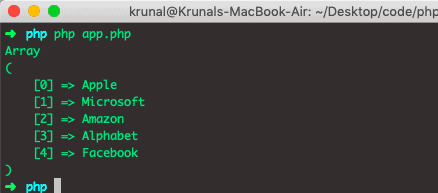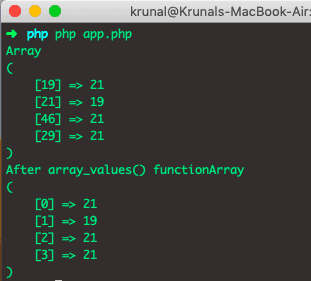# PHP Array Values: How to Get Array Values in PHP

The array_keys() function is used to get an array of values from another array containing key-value pairs or just values.

## PHP Array Values

PHP array_values() is a built-in function that returns all the values of an array and not the keys. The array_values() function returns the array containing all the values of an array. The returned array will have the numeric keys, starting at 0 and increasing by 1.

The PHP array_keys() function creates another array that stores all the values and by default assigns numerical keys to the values.

### Syntax

See the below syntax.

`array_values(array)`

### Arguments

An array is a required parameter, and it specifies the array.

The array_values() function takes only one parameter as a mandatory array and refers to the original input array, from which values need to be fetched.

### Example

See the below code example.

```<?php

// app.php

\$data = ['a' => 'Apple',
'm' => 'Microsoft',
'b' => 'Amazon',
'c' => 'Alphabet',
print_r(array_values(\$data));```

See the output.If you want to fetch all the keys from an array, you can check out the array_keys() function in PHP.

Remember, the PHP array_values() method will ignore your beautiful numeric indexes and renumber them according to the ‘foreach’ ordering.

See the below code example.

```<?php

// app.php

\$data = array(
19 => 21,
21 => 19,
46 => 21,
);
\$data = 21;
print_r(\$data);
echo 'After array_values() function';
print_r(array_values(\$data));```

See the below output.Just a warning that re-indexing an array by array_values() may cause you to reach the memory limit unexpectedly.

The array_keys() and array_values() are very closely related functions. The first function returns an array of all the keys in an array, and the second returns all the values in the array.

For example, consider you had an array with user IDs as keys and usernames as values; you could use array_keys() to generate an array where the values were the keys.

That’s it for this example.

Categories PHP

This site uses Akismet to reduce spam. Learn how your comment data is processed.